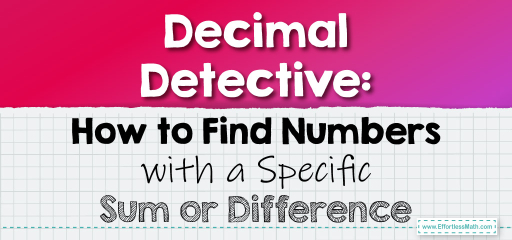# Decimal Detective: How to Find Numbers with a Specific Sum or Difference

In many mathematical and real-world scenarios, we might need to identify or choose decimals that, when added or subtracted, result in a specific sum or difference. This skill is essential for problem-solving and analytical thinking. Let's explore how to approach such problems.## Choosing Decimals with a Specific Sum or Difference

### Example 1: Finding Decimals with a Specific Sum

Which two numbers from the set \{2.5, 3.4, 4.1, 5.6\} have a sum of $$7.9$$?

Solution Process:

1. Start by adding the first number to each of the other numbers in the set.

2. If the sum doesn’t match, move to the next number and repeat.

The numbers $$3.4$$ and $$4.5$$ have a sum of $$7.9$$.

The Absolute Best Book for 5th Grade Students

### Example 2: Identifying Decimals with a Specific Difference

From the set \{8.2, 6.5, 5.7, 4.3\}, which two numbers have a difference of $$1.9$$?

Solution Process:

1. Start by subtracting the first number from each of the other numbers in the set.

2. If the difference doesn’t match, move to the next number and repeat.

The numbers $$8.2$$ and $$6.3$$ have a difference of $$1.9$$.

Choosing decimals with a specific sum or difference requires a systematic approach and careful calculations. By understanding the relationships between numbers and practicing regularly, you can quickly identify the correct pairs of numbers that meet the given criteria. This skill not only enhances your mathematical prowess but also sharpens your analytical thinking, making you adept at solving a wide range of problems with ease!

### Practice Questions:

1. From the set \{1.2, 2.4, 3.4, 4.5\}, which two numbers have a sum of $$5.7$$?

2. Which two numbers from \{7.8, 6.7, 5.6, 4.5\} have a difference of $$2.1$$?

3. From the set \{0.9, 1.8, 2.7, 3.6\}, identify the numbers with a sum of $$4.5$$.

4. Which two numbers from \{9.1, 8.2, 7.3, 6.4\} have a difference of $$0.9$$?

5. From the set \{0.1, 0.2, 0.3, 0.4\}, find the numbers with a sum of $$0.5$$.

A Perfect Book for Grade 5 Math Word Problems!

1. $$1.2$$ and $$4.5$$

2. $$7.8$$ and $$5.7$$

3. $$0.9$$ and $$3.6$$

4. $$9.1$$ and $$8.2$$

5. $$0.1$$ and $$0.4$$

The Best Math Books for Elementary Students

### What people say about "Decimal Detective: How to Find Numbers with a Specific Sum or Difference - Effortless Math: We Help Students Learn to LOVE Mathematics"?

No one replied yet.

X
51% OFF

Limited time only!

Save Over 51%

SAVE $15 It was$29.99 now it is \$14.99